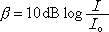Sound Intensity Level beta in terms of Sound Intensity I

For sound, loudness (more often referred to as intensity level or sound intensity level and represented by the Greek letter beta) is equal to 10 decibels times the log of the ratio of the intensity of the sound to the minimum intensity detectable by the human ear.

Note the distinction between sound intensity level (beta) and sound intensity I:

The sound intensity level of some noise is how loud it sounds on a scale of 0 to 100 decibels (and beyond) whereas the sound intensity is the power-per-area of the sound. Interestingly, for sound, if the intensity is increased by a factor of 10 (meaning it becomes 10 times what it was) the intensity level does not increase by a factor of 10; rather, it becomes 10 more decibels than what it was. A multiplicative change in intensity yields an additive change in intensity level. (This is what the equation at the top of this page is telling us.)

You are responsible for knowing that P=IA (power is equal to intensity times area). This is not given to you on your formula sheet, but, if you understand what intensity is, you should be able to deduce this.